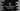# Dart program to get the first n elements from a list## Dart program to get the first n elements from a list:

We can get the first n elements from a list in Dart by using take method. This method takes one value count and it will return a lazy iterable. In this post, we will learn how to use take method to get the first n values from a given list.

### Definition of take:

take method is defined as below:

``````Iterable<E> take(
int count
)``````

This method take one integer value count and returns one lazy iterable holding the first count values of this iterable.

If this list contains less than count number of elements, it will return all the items in the list.

We can iterate through the items of the iterable to get all the values. The count should always a positive value.

### Example of take:

Let’s take a look at the below example:

``````void main() {
var items = [1, 2, 3, 4, 5];
var firstFour = items.take(4);

for (var n in firstFour) {
print(n);
}
}``````

It will print the below output:

``````1
2
3
4``````

We can also convert the iterable to a list directly:

``````void main() {
var items = [1, 2, 3, 4, 5];
var firstFour = items.take(4).toList();

print(firstFour);
}``````

It is converting the first four numbers to a list:

``[1, 2, 3, 4]``